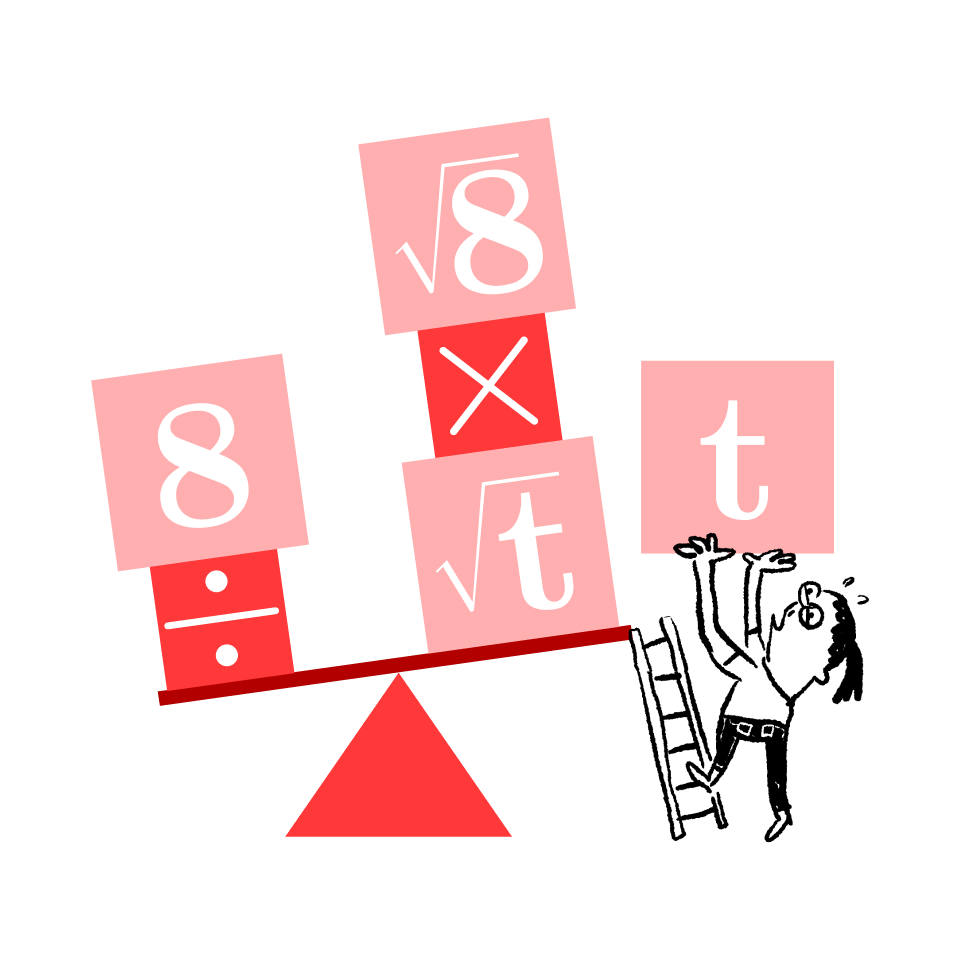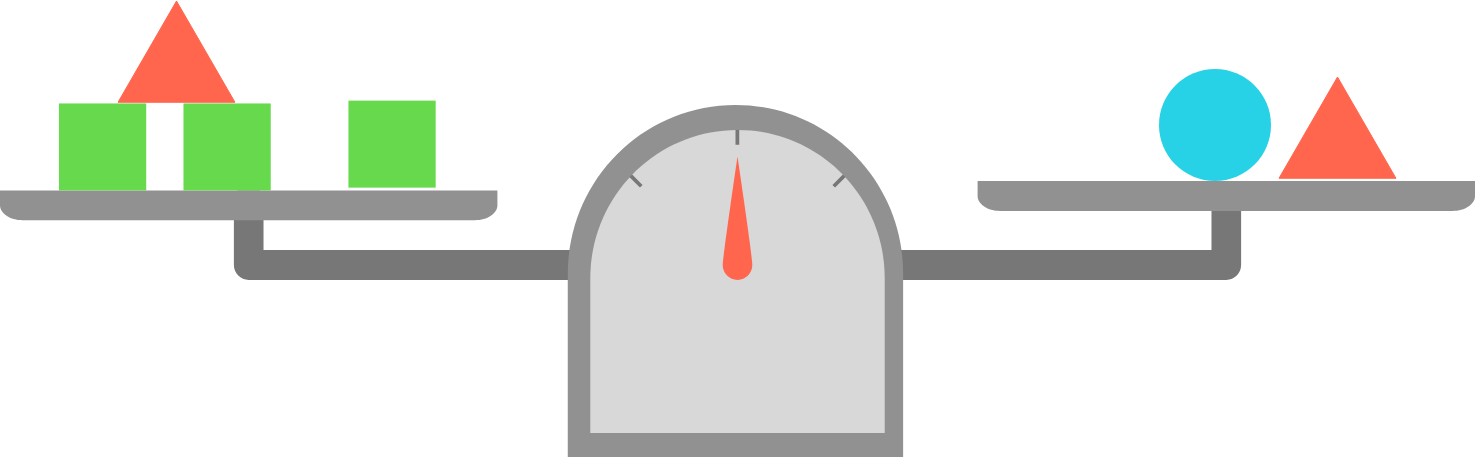Algebra Fundamentals

Scale and Lever Logic

While most approaches to algebra emphasize its mechanical rule-following nature, we will be working to develop an intuition for the principles that make algebra work.In the upcoming problems, try to think about the problems as a physical system that you can manipulate. For example, in the image above, can you imagine what would happen to the scale if you removed only the blue circle? What if you removed both of the red triangles?

Developing this kind of intuition will help you become a stronger problem solver!

×